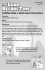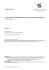# Algorithmic Game Theory Summer 2015 Exercise Set 2

## Transcription

Algorithmic Game Theory Summer 2015 Exercise Set 2
```Xiaohui Bei
Thomas Kesselheim
May 8, 2015
Deadline: lecture on May 18, 2015
Algorithmic Game Theory
Summer 2015
Exercise Set 2
Exercise 1:
(4 Points)
Give an example of a symmetric network congestion game (strategies are s-t paths in a graph
with the same s and t for all players) with non-decreasing delay functions in which there are
at least two pure Nash equilibria with different social costs.
P
The social cost is defined to be the sum of delays of all players i∈N ci (S).
Exercise 2:
(5 + 1 Points)
In a weighted congestion games, each player i ∈ N has an individual weight wi > 0. The
delay on depends on the sum of the weights of the players choosing this resource rather
P than
just on their number. Formally, we could replace the definition of nr (S) by nr (S) = r∈Si wi .
(a) Show that weighted congestion games do not fulfill the finite-improvement property,
even with two players, three resources, and two strategies per player.
Hint: Consider N = {1, 2}, w1 = 1, w2 = 2, R = {a, b, c}, Σ1 = {{a}, {b, c}},
Σ2 = {{b}, {a, c}}. Choose delay functions such that
({a}, {b}) → ({a}, {a, c}) → ({b, c}, {a, c}) → ({b, c}, {b}) → ({a}, {b})
is an improvement sequence.
(b) Why does a solution to (a) imply that there is even no pure Nash equilibrium?
Exercise 3:
(4 + 6 Points)
Load-balancing games are a special case of weighted congestion games. There are m machines
of identical speeds. Player i is in charge of one job of weight wi > 0. Every player may choose
a machine to process this job; his strategy set is therefore [m]. The costs are given by the
time that it takes to process all jobs. That is, player i’s cost in strategy profile s is given as
X
ci (s) =
w i0 .
i0 :si0 =si
(Note that this sum always includes wi .)
(a) Show that there is a strategy profile s that is a pure Nash equilibrium and minimizes
maxi∈N ci (s) at the same time.
(b) Show that load-balancing games fulfill the finite-improvement property.
Hint: Consider the vector of player costs, ordered non-increasingly, and how this vector
changes with respect to the lexicographical order.
```

### Great Skate/ACHA Program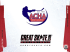### Untitled - West Ham United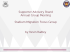### ExamView Player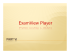### 2015 Registration Flyer - youth football, Campbell County Junior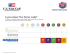### CUSL Roster Instructions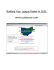### Step by step instructions for players registering for Pelada FA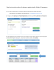### the program flyer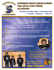### board games online